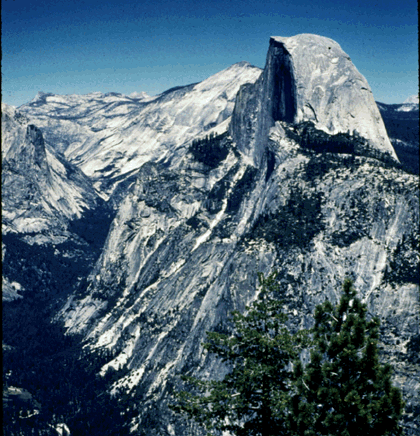## University of California, San Diego Physics 1b - Thermal Physics & Electromagnetism

 H. E. Smith Spring 2000Physics 1B - Tutorial #51. Half Dome in Yosemite National Park has a vertical north face which rises about 1450 m from the floor of Yosemite Valley.
• What is the gravitational potential difference (gravitational potential energy difference per unit mass) between Yosemite Valley and the top of Half Dome.

potential difference = (PEtop - PEbottom)/m = gh = 9.8 m/s2 x 1450m = 14,210 J/kg

• What is the gravitational field near half dome.

g = Fg/m = 9.8 m/s2

• What is the potential gradient down the north face.

potential gradient =(PE/m)/l = gh/h = g

(n.b. Here the potential gradient is just the gravitational field, just as the gradient of the electric potential is the electric field)

• During the summer there are hand railings which allow hikers to climb the south face of half dome. Using the picture above and a ruler or piece of graph paper, estimate the effective potential gradient and gravitational field down the south face.

Here the gradient (slope) is not as steep. If the entire path were the same steepness as the south face of Half Dome, I estimate a path length of 1600m. In this case:

potential gradient =(PE/m)/l = g 1450m/1600m = 0.9g

The effective gravitational field is less.

• What do the lines of equipotential correspond to on a map of Yosemite Valley?

Elevation contours.2. Does a bird sitting on an uninsulated high voltage line get a shock? Explain.

No, and Jennie didn't die at 20,000V. Although the potential of the wire and the bird is high with respect to the ground, the potential difference across the bird is negligible and does no harm. Similarly, it does no harm to raise someone to 30,000 ft. - high gravitational potential. The only harm comes when someone at high potential is connected via a steep path to a region of low potential - someone at 0V touches the bird or you fall from 30,000 ft.

3. Figure (a) below shows measurements of the electrical potential on a grid. Figure (b) shows equipotential contours.• Draw 50V, 70V & 100V contours in Fig. (a).
• If the gridlines in (a) are 1cm apart, estimate the electric field (magnitude and direction) at points A, B, C, & D.

A. E = - dV/dl = 25V/1cm = 2500 V/m (toward left)
B. E = - dV/dl = 50V/1cm = 5000 V/m (toward right)
C. E = - dV/dl = 50V/1.4cm = 3570 V/m (toward upper left)
D. E = - dV/dl = 50V/1.4cm = 3570 V/m (toward lower left)

• In Fig. (b) where is the electric field strongest?

The field is strongest where the contours are closest together - up "1",

• Where is it weakest?

and weakest where the contours are furthest apart - up "2"

• Suppose you were "pushing" a proton from the lowest contour to the highest contour. Which route would you take.

It would be easier, that is require less force, to push a proton up "1",

• Do you save energy by selecting one route over another.

but there is no difference in the work done or the overall change in energy.

• What happens to an electron which starts out at the 0V contour?

"Up hill" to a proton is "downhill" to an electron; it will "fall" to the 8 V contour.

4. A very large conducting plate is charged with 1 C/m2.
• What is the electric field above and below the plate?

E =/20 = 1 C/m2/2 . 8.85 x10-12m2 N-1 C-2 = 5.65 x 1010N/C (away from plate)

• Draw a graph of the electric potential from below the plate to above the plate.

V = Ey

• What is the force on an electron 1 m above the plate?

F = qE = 1.6 x 10-19C . 5.65 x 1010N/C = 9.0 x 10-9N

A second plate, charged with -1 C/m is placed 1cm below the positively charged plate, parallel to it.

• What is the electric field below the lower plate? Between the plates? Above the upper plate.

E = 0 above upper/below lower plate

• Draw a graph of the electric potential from below the lower plate to above the upper plate.
• Now what is the force on the electron?

F = 0

Gene Smith